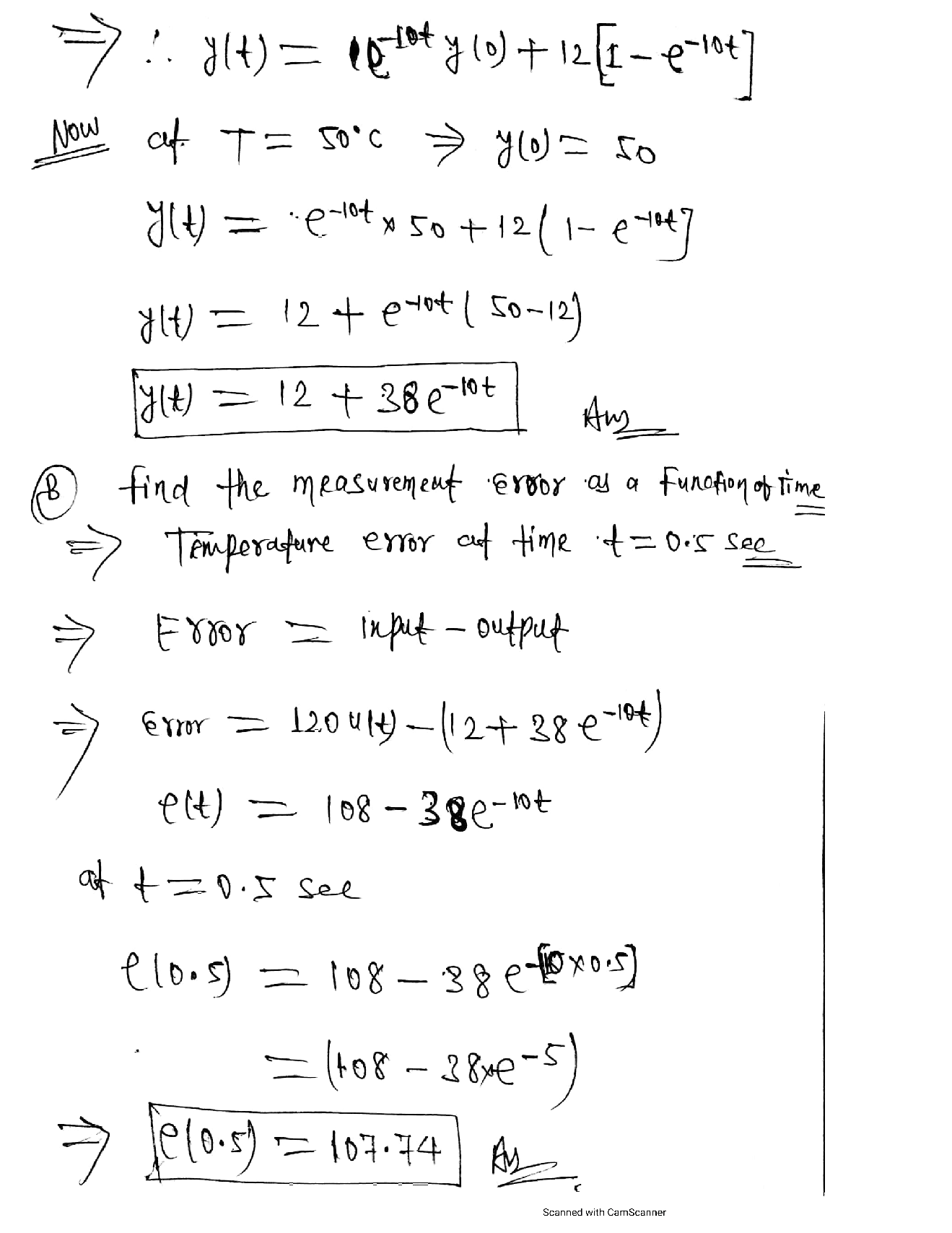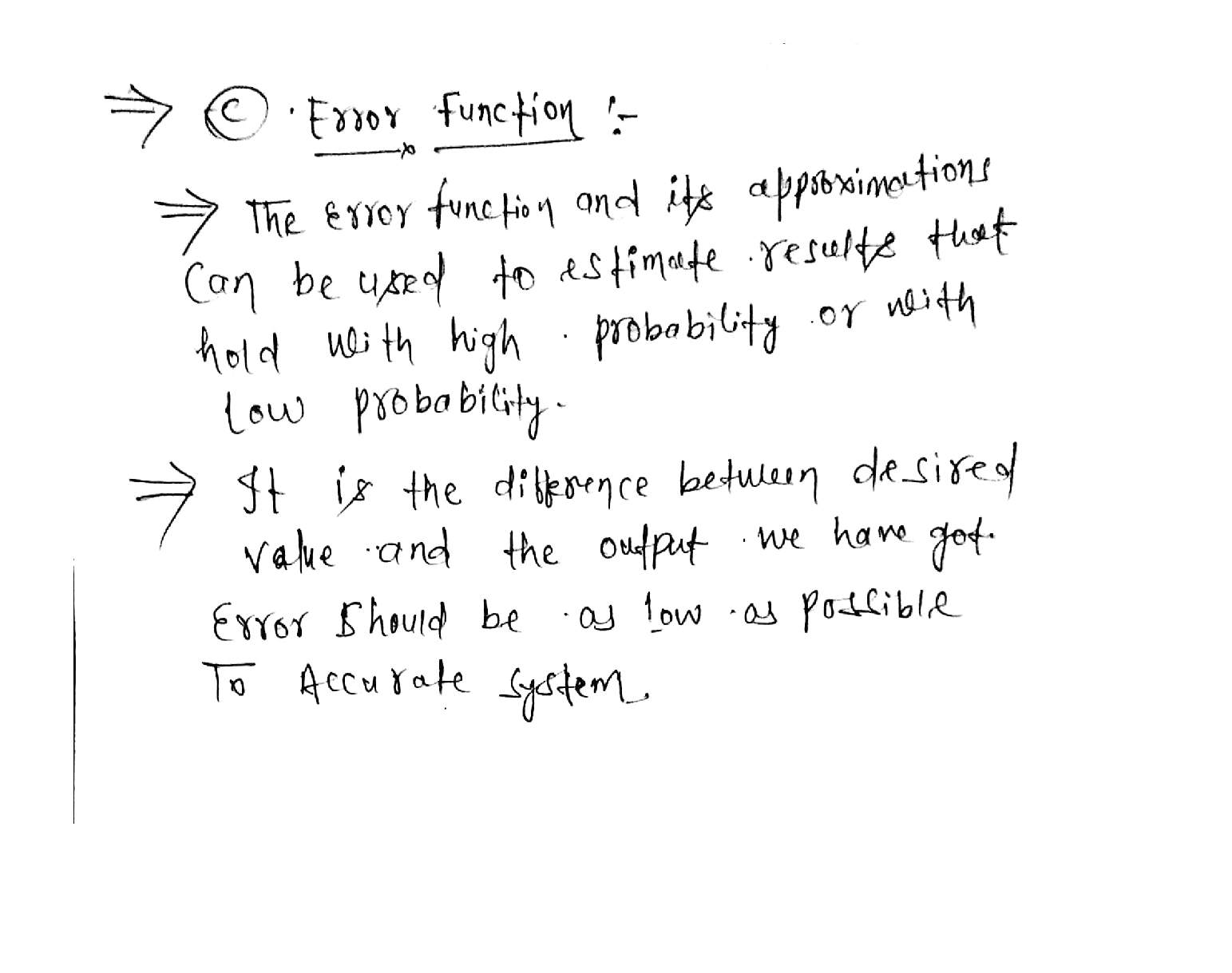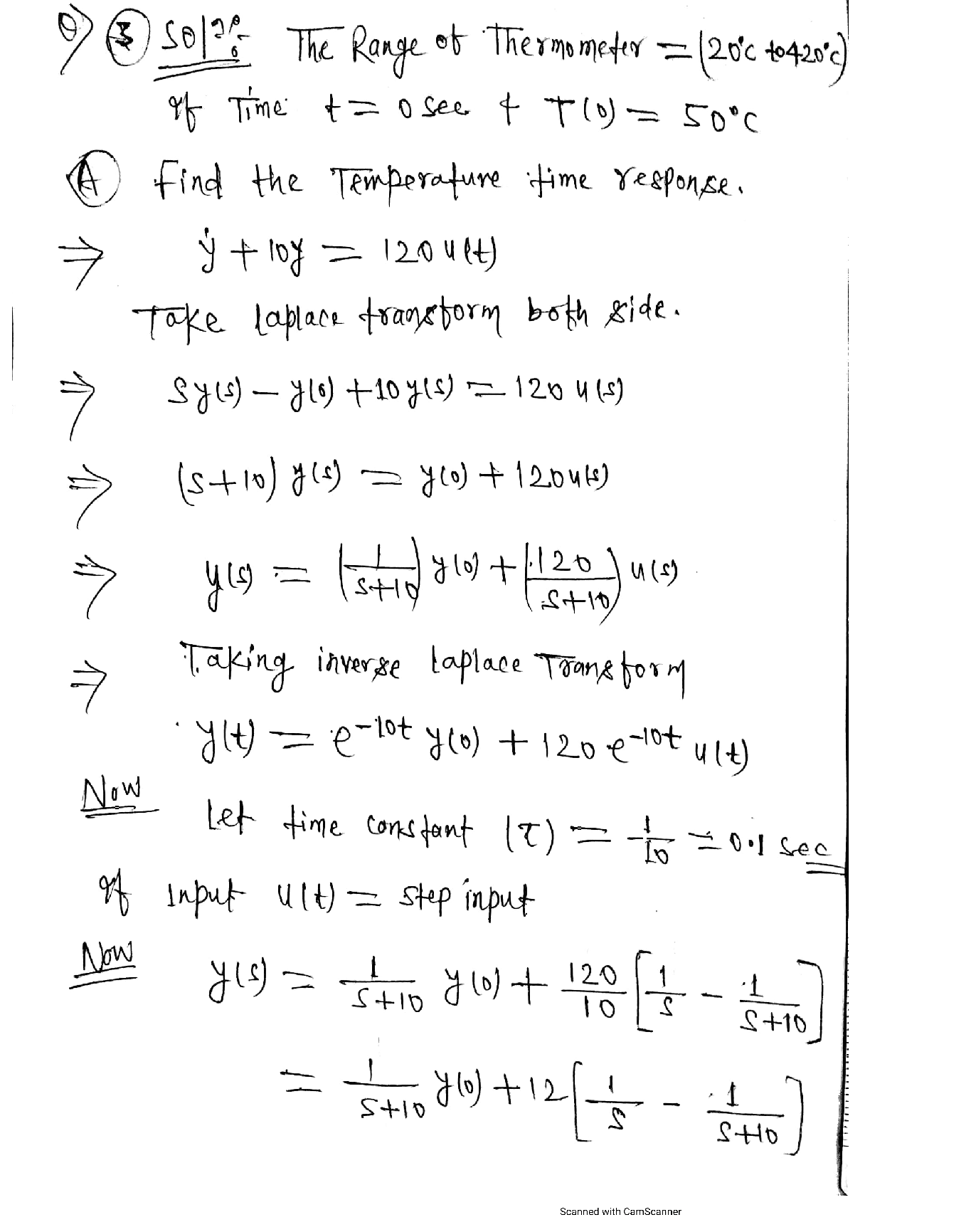Question

Signals

A thermometer with a first-order dynamic is used for a test. The range of thermometer is20°c-420°c. If, at time t=0s, T(0)= 50°c

a) Calculate the temperature time response of the sensor if ý + 10y =120U(t).

b) Find the measurement error as a function of time, e(t), and calculate the measuring temperature error at time t=0.5sec. (5 points)

Explain the error fraction in your own words (5 points)Verified### Question 45411Signals

Question II: Figure (2) shows the function g(t), draw the function g(-3t+4.5) showing the calculations for the points in the graph.(7.5 Marks)

### Question 45410Signals

I: For the signal g(t) shown in figure (1) below:
a. Determine the period of the signal g(t). (1 Mar
b. Determine if g(t) represents power or energy signal and then calculate the value of it.(6.5 Morlca)

### Question 42955Signals

Q4) A radio transmitter operated at f0 MHz has an internal impedance of ZG N and it delivers power of Pdel W to a matched load. It is connected to an antenna of impedance ZL Q via a loss-free ZO Q coaxial cable with length of L m. Find
a) The The venin equivalent of the transmitter together with the transmission line, i.e., with respect to load terminals.
b) The power delivered to the antenna.
c) The power reflected back to the generator.

### Question 42954Signals

Q3) The results of a slotted-line experiment are plotted in the following figure. The length of the line is & cm; its characteristic impedance is Z0 Q.
b) The load impedance.
d) The reflection coefficient at the generator terminals.
a) The reflection coefficient at the load.
c) The input impedance.

### Question 42953Signals

) For the T network shown below, R1 and R2 given on the table. Find
a) The scattering matrix
b) The reflected-to-incident power ratio.
c) The transmitted-to-incident power ratio.
d) The loss-to-incident power ratio.
e) What is the function of this device?

### Question 41049Signals

2. Consider Amplifier and filter system

### Question 41048Signals

Problem 1. Determine the Discrete-time Fourier Transform of A squarewave is
x[n]=(0.5)^{n} u[n]
\text { Plot the magnitude and phase of } H(\Omega) \text { in matlab. }

### Question 40577Signals

A silicon sample is in equilibrium at T = 400k. It is given that the hole concentration of po =7.1 x 1016 cm-3 at this temperature.
What is the concentration of electrons?
| What is the type of the semiconductor? Justify your answer
[10 Points] Where is the Fermi level located with respect to the intrinsic level? (A numerical answer is required)

### Question 40576Signals

Consider a substrate of silicon at T = 300 K is doped with arsenic atoms (donors) having a concentration of 3× 1015 cm-3 and with boron atoms (acceptor) having a concentration of3 x 1014 cm-3. Consider that Np and NA are not affected by temperature.
[15 Points] At two different temperature points T = 300 K and T =700K, Is the material n- or p- type semiconductor? Justify your answer and explain the effect of the temperature on this.
| Does temperature affect the Energy Fermi-Level? Justify your answer

### Question 40575Signals

The energy band diagram of germanium shows the location of a particular energy levelE, at T =600 K.
[10 Points] What is the type of semiconductor represented in the energy diagram?Justify your answer.
Calculate the number of free electrons at energy level E,.
| Calculate the intrinsic carrier concentration.
| If Na is considered as zero, calculate the number of Donor atoms (ND).
Calculate the total number of free electrons.

### Submit query

Getting answers to your urgent problems is simple. Submit your query in the given box and get answers Instantly.

### Submit a new QuerySuccess

Assignment is successfully created